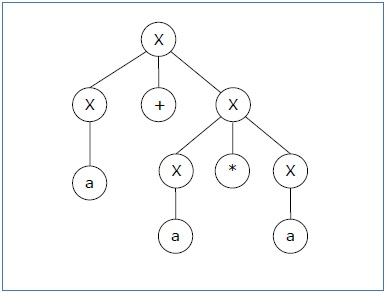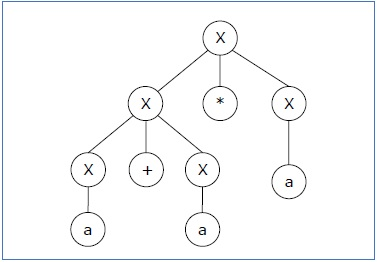# Ambiguity in Context-Free Grammars

If a context free grammar G has more than one derivation tree for some string w ∈ L(G), it is called an ambiguous grammar. There exist multiple right-most or left-most derivations for some string generated from that grammar.

## Problem

Check whether the grammar G with production rules −

X → X+X | X*X |X| a

is ambiguous or not.

## Solution

Let’s find out the derivation tree for the string "a+a*a". It has two leftmost derivations.

Derivation 1X → X+X → a +X → a+ X*X → a+a*X → a+a*a

Parse tree 1Derivation 2X → X*X → X+X*X → a+ X*X → a+a*X → a+a*a

Parse tree 2Since there are two parse trees for a single string "a+a*a", the grammar G is ambiguous.